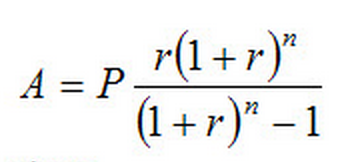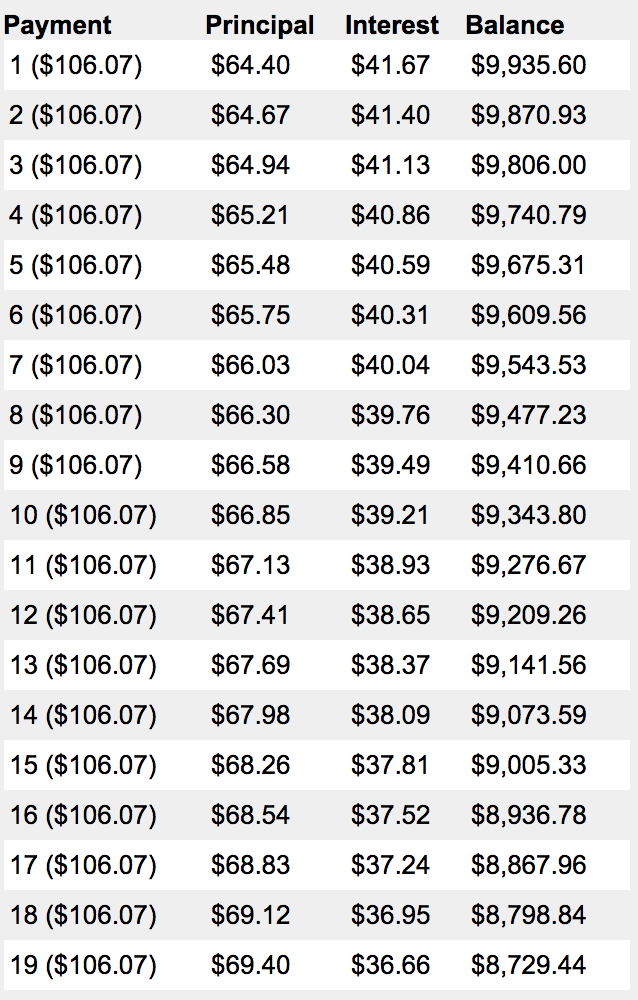# What is amortization? Definition and examples

Amortization is a term people commonly use in finance and accounting. However, the term has several different meanings depending on the context of its use.

Amortization may refer the liquidation of an interest-bearing debt through a series of periodic payments over a certain period. In most cases, the payments over the period are of equal amounts. Paying in equal amounts is actually quite common when taking out a loan or a mortgage.

According to Oxford Dictionaries, the verb ‘to amortize’ is to “gradually write off the initial cost of (an asset) over a period.”

In accounting, amortization refers to the assignment of a balance sheet item as either revenue or expense. You can find the item on the income statement.

Amortization also refers to a business spreading out capital expenses for intangible assets over a certain period. In fact, accountants do this for tax and accounting reasons. By amortizing certain assets, the company pays less tax and may even post higher profits.

In fact, in this sense, the meaning is similar to **depreciation. The accountant is estimating an asset’s expense with how much revenue it brings in.

** Depreciation is an accounting word that describes how long-term assets decline in value over time. Most assets decline in value because of wear and tear. However, some do not.

## Amortization of loans

An amortization schedule determines the distribution of payments of a loan into cash flow installments. As opposed to other models, the amortization model comprises both the interest and the principal.

Amortization is one of the simplest repayment models there are. It is very simple because the borrower pays the repayments in equal amounts during the loan’s lifetime.

Typically, more money is applied to interest at the start of the schedule. Towards the end of the schedule, on the other hand, more money is applied to the principal.

We amortize a loan when we use a part of each payment to pay interest. Subsequently, we use the remaining part to reduce the outstanding principal.

Over time, after the series of payments, the borrower gradually reduces the outstanding principal. Additionally, interest on the unpaid balance falls.

Once a debt is amortized by equal payments at equal intervals, the debt becomes an annuity’s discounted value.

We use amortization tables to represent the composition of periodic payments between interest charges and principal repayments.

Negative amortization can occur if the payments fail to match the interest. In this case, the lender then adds outstanding interest to the total loan balance. As a consequence of adding interest, the total loan amount becomes larger than what it was originally.

### The Amortization Schedule Formula:A = payment amount per period. P = initial principal (loan amount). r = interest rate per period. n = total number of payments or periods.

## Calculating the amortization of a loan

You can calculate a loan’s payoff if you know:

– The starting amount of the loan.

– And also how many payments you will need to make.

An amortization table provides you with the principal and interest of each payment.

Assume that you have a ten-year loan of \$10,000 that you pay back monthly. Also, assume that the annual percentage interest rate on this loan is 5%.

### Step 1: How many repayments will you make?

In the example above, the loan is paid on a monthly basis over ten years. Therefore there is a total of (12 x 10) 120 loan payments.

### Step 2: Calculate the period interest rate.

To calculate the period interest rate you divide the annual percentage rate by the number of payments in a year.

In the example, payments are made on a monthly basis. Therefore, there are 12 payments each year. The annual interest rate is 5%. Therefore, the period interest rate would be (0.05/12) = 0.0041666, or 0.41667%.

### Step 3: Use the Amortization Schedule Formula

You can use the amortization schedule formula to calculate the payment for each period.

In our example, the loan amount is \$10,000. Our period interest rate is 0.00417. Our total number of payments is 120.

Amount per period = (10,000)*[(0.004166)((1+0.004166)^120)]/[(((1+0.004166)^120) -1)]

= \$106.07

### Step 4: Draw a table.

Draw three columns. Give the first column the title of ‘Current Value’. Call the second column ‘Interest’, and the third column ‘Principal’.

In this example, you would write \$10,000 at the top of the Current Value column.

### Step 5: Calculate the Interest and Principal values and add them to your table

Multiply the current loan value by the period interest rate to get the interest. Then subtract the interest from the payment value to get the principal.

The “Interest” in the example would be: \$10,000 * 0.00417 = \$41.7

The Principal would be: \$106.08 – \$41.7 = 64.40

### Step 6: Subtract the principal from the current value and write the new current value in the second row of the ‘Current Value’ column.

\$10,000 – 64.40 = \$9935.60

### Step 7: Repeat steps 5 and 6.

In our example, the table would look like this. N.B. the table is not complete and only shows up to the 19th payment:## Amortization of intangible assets

Amortization also refers to the acquisition cost of intangible assets minus their residual value. In this sense, the term reflects the asset’s consumption and subsequent decline in value over time.

Amortization does not relate to some intangible assets, such as goodwill. Some intangible assets do not have a set period of ‘life’.

We record the amortization of intangible assets in the financial statements of a company as an expense.